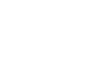What is Newton-s third law? - letsdiskussTeja Swi

Student | Posted | Science-Technology

# What is Newton-s third law?

0
0

| Posted

A force applied to a body can change the magnitude of the momentum, or its direction, or both.  Newton's third law states that when two bodies interact, they apply forces to one another that are equal in magnitude and opposite in direction. The third law is also known as the law of action and reaction.

0
0

student | Posted

The great scientist and physicist, Newton had been provided us three laws of motions. The Third law of motion is stated as- " If an object A exerts a force on other object B, then the object B must exert an equal and opposite force on object A". This force of object B on object A must be equal in magnitude and opposite in direction to the force of object A on object B. This law describes that "For every action, there is an equal and opposite reaction".0
0

designer | Posted

The third law states that when one object exerts a force on a second object, that second object exerts a force that is equal in magnitude and opposite in direction on the first object.

0
0